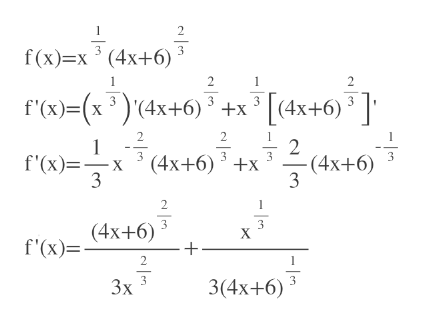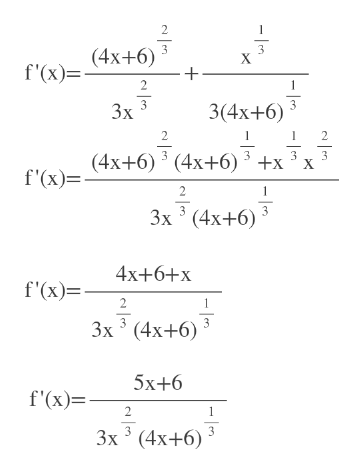# Let f (x) = x1/3(4x + 6)2/3.   Find f ' (x) and the critical numbers for f.f ' (x) = Ax + B3 xC (4x + 6)D, whereA = B = C = D = The critical numbers of f are (list in increasing order):x = x = x =(Enter DNE in any unused answer blank.)

Question
1 views
Let f (x) = x1/3(4x + 6)2/3.   Find f ' (x) and the critical numbers for f.

f ' (x) =
 Ax + B 3 xC (4x + 6)D
, where
A =
B =
C =
D =

The critical numbers of f are (list in increasing order):
x =
x =
x =

(Enter DNE in any unused answer blank.)

check_circle

Step 1

Use product rule to find f'(x).help_outlineImage Transcriptionclose2 f(x)=x3 (4x+6) 2 2 f'(x)=(x3)(4x+6)3 x (4x+6) 3 2 2 1 2 (4x+6)x 3 f'(x)=X 3 (4x+6) 2 (4x+6) X f'(x)= 2 3 3x 3(4x+6) fullscreen
Step 2

Simplify it using LCDhelp_outlineImage Transcriptionclose2 3 X (4x+6) f'(x)= 2 Зx 3(4x+6) 2 (4х+6) ' (4х+6)* +x *x 3 f'(x)= 2 Зх 3 (4x+6) 4x+6+х г')- 2 Зх (4х+6) 3 5х+6 f'(x) 2 Зх 3 (4х+6) - |. fullscreen

### Want to see the full answer?

See Solution

#### Want to see this answer and more?

Solutions are written by subject experts who are available 24/7. Questions are typically answered within 1 hour.*

See Solution
*Response times may vary by subject and question.
Tagged in

### Other